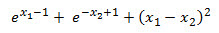# SQL Server BFGS function

BFGS
Updated: 16 April 2018

Use the scalar function BFGS to find the minimum of a functions using the Broyden-Fletcher-Goldfarb-Shanno (BFGS) method.

Syntax
SELECT [wct].[BFGS] (
<@Func, nvarchar(max),>
,<@VarNames, nvarchar(4000),>
,<@X, nvarchar(4000),>
,<@H, float,>
,<@tol, float,>)
Arguments
 Input Name Description @Func The function to be evaluated, as a string. The function must be in the form of a SELECT statement. @VarName The name of the variable @X The starting point for the minimization @H Step size @tol Tolerance (for the solution)
Return Type
[nvarchar](max)
Remarks
• If @Func returns a NULL then NULL Is returned.
• If @Func is not a valid SELECT statement then NULL is returned.
• If no solution is found then NULL is returned.
• If @X is NULL then @X = ''.
• If @H is NULL then @H = 0.
• If @H <= 0 then @H = 2^(-52).
• If @tol is NULL then @tol = 0.0001
• Available in XLeratorDB / math 2008 only
Examples
Example #1

Calculate the minimum ofOur initial estimate is the point (0,0).

Since the result is returned as a string, we will use the MATRIX function to unpack the results into a vector format.

SELECT
*
FROM
wctMath.wct.MATRIX(
wct.BFGS(
'SELECT EXP(@x1-1) + EXP(-@x2+1) + POWER(@x1 - @x2,2)'
,'@x1,@x2'
,'0,0'
,NULL
,NULL)
)

This produces the following result.Copyright 2008-2021 Westclintech LLC         Privacy Policy        Terms of Service